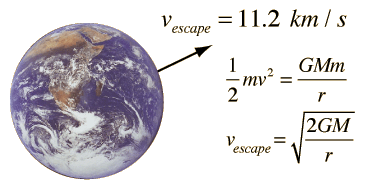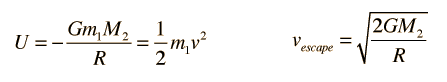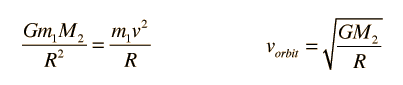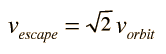# Escape Velocity

If the kinetic energy of an object launched from the Earth were equal in magnitude to the potential energy, then in the absence of friction resistance it could escape from the Earth.
 Escape velocity from the EarthIf M = MEarth

 and r = rEarth

 then vescape = m/s

vescape =km/hr
vescape =mi/hr.

This data corresponds to a surface gravitational acceleration of

g = m/s2
g = gEarth.
 Orbit velocity and escape velocity
Index

Energy concepts

Gravity concepts

 HyperPhysics***** Mechanics R Nave
Go Back

# Orbit Velocity and Escape Velocity

If the kinetic energy of an object m1 launched from a planet of mass M2 were equal in magnitude to the potential energy, then in the absence of friction resistance it could escape from the planet. The escape velocity is given byTo find the orbit velocity for a circular orbit, you can set the gravitational force equal to the required centripetal force.Note that the orbit velocity and the escape velocity from that radius are related byIndex

Energy concepts

Gravity concepts

 HyperPhysics***** Mechanics R Nave
Go Back